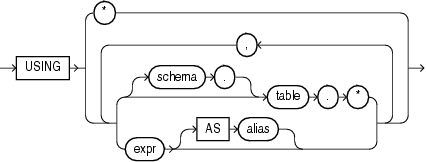PREDICTION_BOUNDS

SyntaxDescription of the illustration prediction_bounds.eps

mining_attribute_clause::=Description of the illustration mining_attribute_clause.eps

Purpose

PREDICTION_BOUNDS applies a Generalized Linear Model (GLM) to predict a class or a value for each row in the selection. The function returns the upper and lower bounds of each prediction in a varray of objects with fields UPPER and LOWER.

GLM can perform either regression or binary classification:

• The bounds for regression refer to the predicted target value. The data type of UPPER and LOWER is the data type of the target.

• The bounds for binary classification refer to the probability of either the predicted target class or the specified class_value. The data type of UPPER and LOWER is BINARY_DOUBLE.

If the model was built using ridge regression, or if the covariance matrix is found to be singular during the build, then PREDICTION_BOUNDS returns NULL for both bounds.

confidence_level is a number in the range (0,1). The default value is 0.95. You can specify class_value while leaving confidence_level at its default by specifying NULL for confidence_level.

The syntax of the PREDICTION_BOUNDS function can use an optional GROUPING hint when scoring a partitioned model. See GROUPING Hint.

mining_attribute_clause

mining_attribute_clause identifies the column attributes to use as predictors for scoring. This clause behaves as described for the PREDICTION function. (Note that the reference to analytic syntax does not apply.) See .

Note:

The following example is excerpted from the Data Mining sample programs. For more information about the sample programs, see Appendix A in Oracle Data Mining User's Guide.

Example

The following example returns the distribution of customers whose ages are predicted with 98% confidence to be greater than 24 and less than 46.

SELECT count(cust_id) cust_count, cust_marital_status
FROM (SELECT cust_id, cust_marital_status
FROM mining_data_apply_v
WHERE PREDICTION_BOUNDS(glmr_sh_regr_sample,0.98 USING *).LOWER > 24 AND
PREDICTION_BOUNDS(glmr_sh_regr_sample,0.98 USING *).UPPER < 46)
GROUP BY cust_marital_status;

CUST_COUNT CUST_MARITAL_STATUS
-------------- --------------------
46 NeverM
7 Mabsent
5 Separ.
35 Divorc.
72 Married